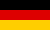Bri's worldelectronics, programming and more

deutschThis web app calculates the resistors for an adjustable voltage divider. The resistors are selected from E12 or E24 resistor series.

 Volt 1: Volt 2 minimum: Volt 2 maximum:
 Volt 1 minimum: Volt 1 maximum: Volt 2:
 current I: mA Resistor series: E12 E24 E48 E96 Potentiometer series:

Result calculated

R1 Rp R2 min. voltage max. voltage

Result selected resistors

R1 Rp R2 min. voltage max. voltage min. current max. current